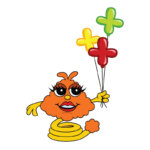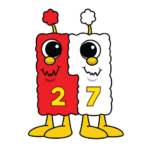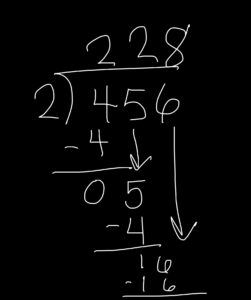Select Page

# Shannon’s Sequence of Division Strategies

Nov 22, 2019

Division seems to be a hot topic right now as we’re working with our schools.In third grade, the standards have students start learning the foundations of division and dividing groups as it relates to arrays and multiplication. As students get into fourth and fifth grade, they start learning different strategies to help with division. While most of us learned division the traditional way, it’s really still appropriate to learn division with number sense in a way that has students attending to do place value BEFORE they we teach the traditional strategy with T-Pops.

I saw the power of teaching different division strategies first-hand when my 5th grade son, Connor, came home about a month ago really confused about long division. He told me that he understood the concept, but didn’t see why people drew arrows to move numbers down when they don’t actually represent that certain value.

The sequence that I took Connor through to answer his question is the way that I would teach division to fourth and fifth grade students to help them solidify an understanding of what’s going on when we divide, even if we’re using the traditional method.

## Conceptual Understanding with Place Value Discs

To begin understanding division, we must start with a conceptual understanding of what it looks like and what kind of language we use. In multiplication, we say, “There are five groups of seven, how many in all?” For division, we might reverse that statement and say, “How many groups of seven are in 35?” We want kids to understand that we’re dividing up our target number into groups and we want to see just how many there are within that target number.

Using place value discs, we can establish that conceptual understanding that we need. In this example, let’s use 48 ÷ 4. I would build it with four tens and eight ones, either on a mat or somewhere kids could maybe write with dry erase markers, then ask the students, if we divided 48 into four groups, how many would be in each group? We could then start to divide the discs evenly into four groups on the mat. I’d start with giving each group a ten discs, and then each group gets a one disc, and then distribute the remaining discs evenly until they were gone. Kids could then see that, within each group, there were 12, so the answer would be 12 groups of four in the number 48. We could also do this with a number like 49 to help kids visualize remainders.

When using place value discs, make sure you choose problems with reasonable quantities so you have enough discs per student! You don’t want to use a number so large that you have to combine sets. You also don’t want a quantity that will take so long to distribute that students lose focus and fail to grasp the concept of division.

We want kids to see what division looks like as you divide up the discs. Our Why Before How book, by Jana Hazekamp, is a great resource to help you show the reasoning behind the concepts, and we highly recommend it as a companion to using place value discs to help students gain an understanding of what division really is.

This conceptual understanding of division is the foundation upon which students can build as they learn new strategies for division or work with larger divisors or dividends. Students can also relate division to their understanding of multiplication, and we try to anchor their number sense and use friendly numbers that kids can kind of grab onto.

In addition to the videos I’ll share here, there are some other great video resources about division here at LearnZillion.

## Multiplying Up

The first strategy in the sequence is called multiplying up, and not all textbooks use this strategy. Some go right into the traditional method, which confuses kids very quickly because they’re being presented with a procedure to memorize for a concept they don’t understand yet.

Multiplying up involves using what you already know to guess-timate how many groups of that number are in a target number.

Let’s take 364 divided by 4. Our target number is 364, and we need to figure out how many would be in 4 groups if we divided that number evenly.

I set up the problem with a line for the number that we’re going to estimate to think about how many times it goes into the target number, and I put a box around the target number. Then, we begin to take an inquiry-based approach, which is strongly supported by this strategy. This helps students to understand precision and reasoning when they’re thinking about division.

We can begin with an estimate – could there be at least 100 groups of four in our target number? Does that even make sense to students? We want them to respond with thoughts, not just information. So let them grapple with their thoughts. Let them talk about it in groups. Could there be 100 groups of four in 364 – why or why not?

We might get an answer like, “That wouldn’t be possible because if we take 100 x 4, that’s 400, which is more than 364.” Prior to doing division, kids have done a lot of things in multiplication. They can “times” something by 10 or by 100, so those benchmarks are familiar. After they determine that 100 is too much, I might guide them down to the next benchmark of 50. “Do you think there are at least 50 groups of 4 in 364?” Kids would respond, “I know that 50 times 4 is  200.” So we write down 50 groups of 4, and write 200 down as the total. Are we at 364? Not yet!

We know another 50 groups won’t work because we already said that 4 times 100 is too much. Let’s try another friendly number. I know 25 groups of 4 is 100, so I write that down and add up the two totals (200 + 100) to get 300. We’re getting closer, but not quite at that target number yet. Another 10 groups of 4 would add another 40, so we’d have 340 in all. We can go to the next friendly number and do 5 groups of 4, which is 20. Adding it together, we end up with 360. Just one more group of 4 will help us hit the target number.

We then go back to add up how many groups we used – 50 + 25 + 10 + 5 + 1, which equals 91 groups of 4. I put a circle around the number of groups to indicate our answer – 91.The magic in this strategy is that we are really thinking about the steps we’re taking as we multiply up to get to our target number. We can think about Springling with this strategy because she’s multiplying up in groups. Instead of hopping up the number line, she can hop up in groups, say 50 groups of 4, then 20 groups of 4, and so on until she reaches the target number.

Multiplying up helps the students catch those benchmark numbers and helps them take the number and keep bringing it down to the ballpark estimate of their target number. Eventually, they drill down and figure out how many actual groups are in the number. You could also use this strategy with remainders. The only difference would be that, after students have drilled down to many groups are in the target number, whatever is left is the remainder.

## Partial Quotients

The next strategy, partial quotients, moves into traditional algorithms of sorts. Partial quotients is really taking the strategy of multiplying up but putting the numbers into a standard algorithm so kids can make the connection to division. We put the problem into the traditional division bracket, except it has a long tail which creates a place for students do their computation and show totals off to the side.

Let’s do 481 ÷ 5. Of course you could do with with any different dividend or divisor, depending on how high you want to go with your students, especially as their number sense gets stronger. You’d still use those ballpark estimates, even dividing by two- or three-digits numbers, and build from there.

Looking at how many groups of 5 are in 481, we’ll put the problem into the division bracket and draw a line going to the side so we can keep our running totals.

We’ll put our groupings off to the side, as we did before. We already know that 100 times is too many, so we can start with the friendly number of 50 groups of 5. So, we put 50 off to the side of the tail and then multiply 50 times 5, which is 250. Write that number under the target number so students can see how they’re subtracting within the algorithm and keep a running total as they’re starting to dwindle that number down. 481 – 250 is 231, so how many groups of 5 can go into the amount that’s left?

We can see that we can’t do another 50, but maybe we think of trying 40 groups of 5. So, we write 20 off to the side under the 50.  40 x 5 is going to knock 200 off the total we’re working with. So 231 becomes 31.From there, the kids might decide to try 5 groups of 5 or start skip counting by 5s – 5, 10, 15, 20, 25, 30 – to see that there are 6 groups of 5 within the running total of 31. Off to the side, we have 50 groups of 5, 20 groups of 5, 20 groups of 5, and then 6 groups of 5. When you subtract 30, you end up with a remainder of 1, and we know that we can’t put another group of five into 1. We can add 50, 20, 20, and 6 to find out that, within 481, there are 96 groups of 5 with a remainder of 1

In some ways, we could relate partial quotients to Value Pak because we’re using the actual values of the numbers as we investigate the number of groups.

If kids understand how to multiply up, and then how to do partial quotients, they can begin to see how division looks in a traditional algorithm.  But instead of using “shortcuts” like arrows to “bring numbers down,” like we learned when we were taught the traditional, which devalues the numbers and can be really confusing to students, we want to approach it a little differently at first.

Our example for this strategy will be 256 ÷ 4.In the traditional method, you might approach the problem by asking does 4 go into 2? This is not good practice because that 2 is not actually a 2. The value of it is 200, and yes, 4 does go into 200 quite a few times. But we can’t write a 1 above the bracket because that 1 would really represent 100, and 100 times 4 is 400, which is higher than our target number.

When looking at the traditional algorithm, we really want to refrain from saying a number doesn’t “go into” another number, because it actually might when you look at the value! The better we can help kids to understand that concept and attend to place value, when they start to learn the “shortcut version” later on, they’ll actually understand what they’re doing. It will even help them when they get to algebra and the values they’re working with might be X.

In our example, we can go to the next number and see that 25 is really 250, so we must find out how many groups of 4 are in 250. Let’s try 60 groups of 4 to see if that will fit into 250. Most kids would just write the 6 above the bracket, do the multiplication of 60 x 4 (240), then subtract and draw an arrow to bring the last digit down. Really attending to place value, though, that 6 written on top of the bracket isn’t worth 6, it’s actually worth 60. When we subtract 240 from 256, we’re left with 16. Now we’re asking how many groups of 4 are in 16. We know that 4 groups of 4 is 16, so, we write the 4 on the bracket, subtract the totals, and we’re left with 0. Done!Yes, in the end, drawing the arrows down is a nice shortcut, but if we jump to that strategy without the foundation behind it (multiplying up, then partial quotients, then the traditional method with partial quotients within the algorithm), it will completely confuse our students. We can introduce shortcuts once the foundations of number sense have been firmly established.